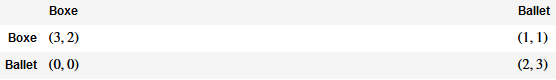# Table intercolumn spaces

This code

A = matrix([[3, 1], [0, 2]])
B = matrix([[2, 1], [0, 3]])
bots = NormalFormGame([A, B])
botsr=[[tuple(bots[(0,0)]),tuple(bots[(0,1)])],[tuple(bots[(1,0)]),tuple(bots[(1,1)])]]
show(t)


produce this. is ther a way to shorten the space between columns because it's ugly

edit retag close merge delete

Indeed there is no problem in SageCell. But with SageMath version 9.7 with docker there is a problem

Sort by » oldest newest most voted

In sage 9.8 the workaround works for me:

A = matrix([[3, 1], [0, 2]])
B = matrix([[2, 1], [0, 3]])
bots = NormalFormGame([A, B])
botsr=[[tuple(bots[(0,0)]),tuple(bots[(0,1)])],[tuple(bots[(1,0)]),tuple(bots[(1,1)])]]
import pandas as pd
jpm=pd.DataFrame(botsr, columns=['Boxe', 'Ballet'])
jpm.index = ['Boxe', 'Ballet']
jpm

more

Of course it works also for me. But you use Pandas. Because of that I hesitate to say that your answer is a good one. I think there is a bug in Sagemath Table at least for v.9.7